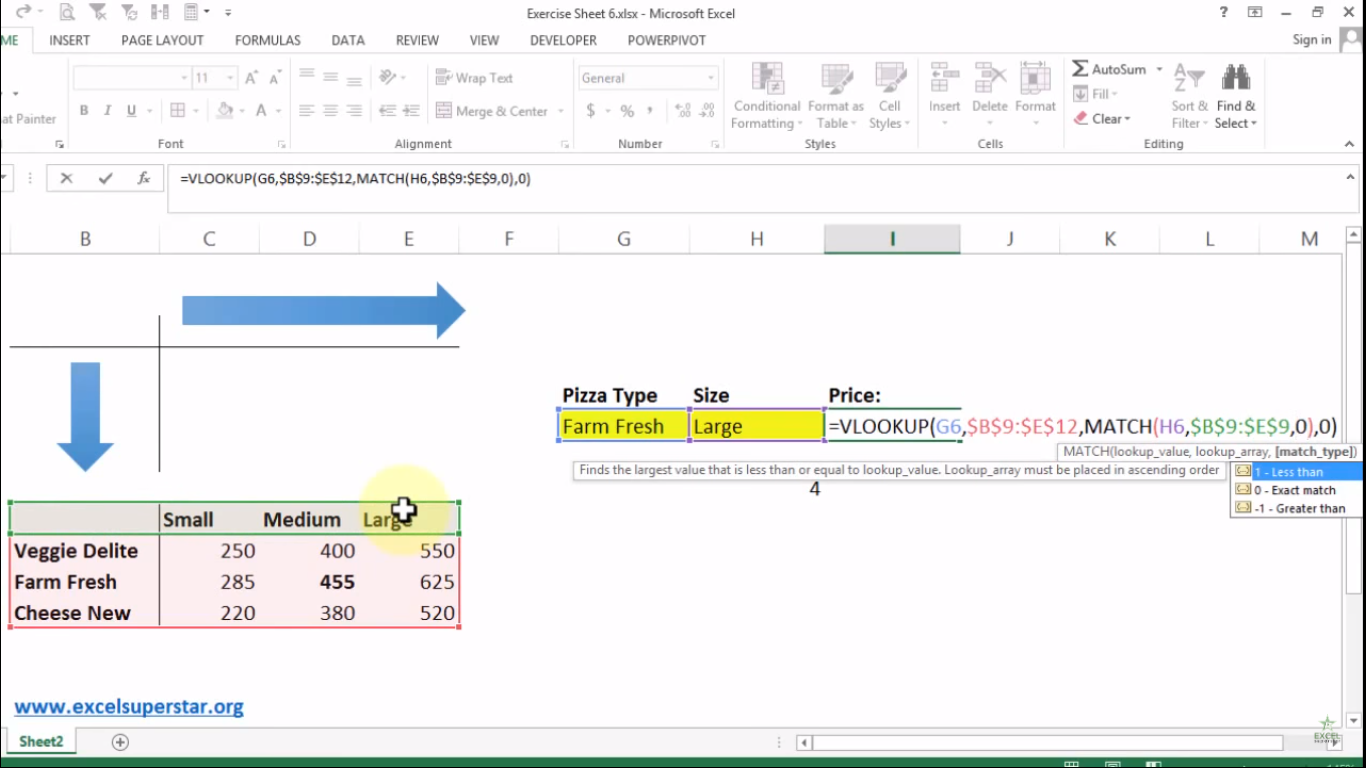# Excel VLOOKUP Formula with MATCHIn this tutorial we will learn about Using VLOOKUP with MATCH for 2d lookup in Excel with the help of an example given below:

Example: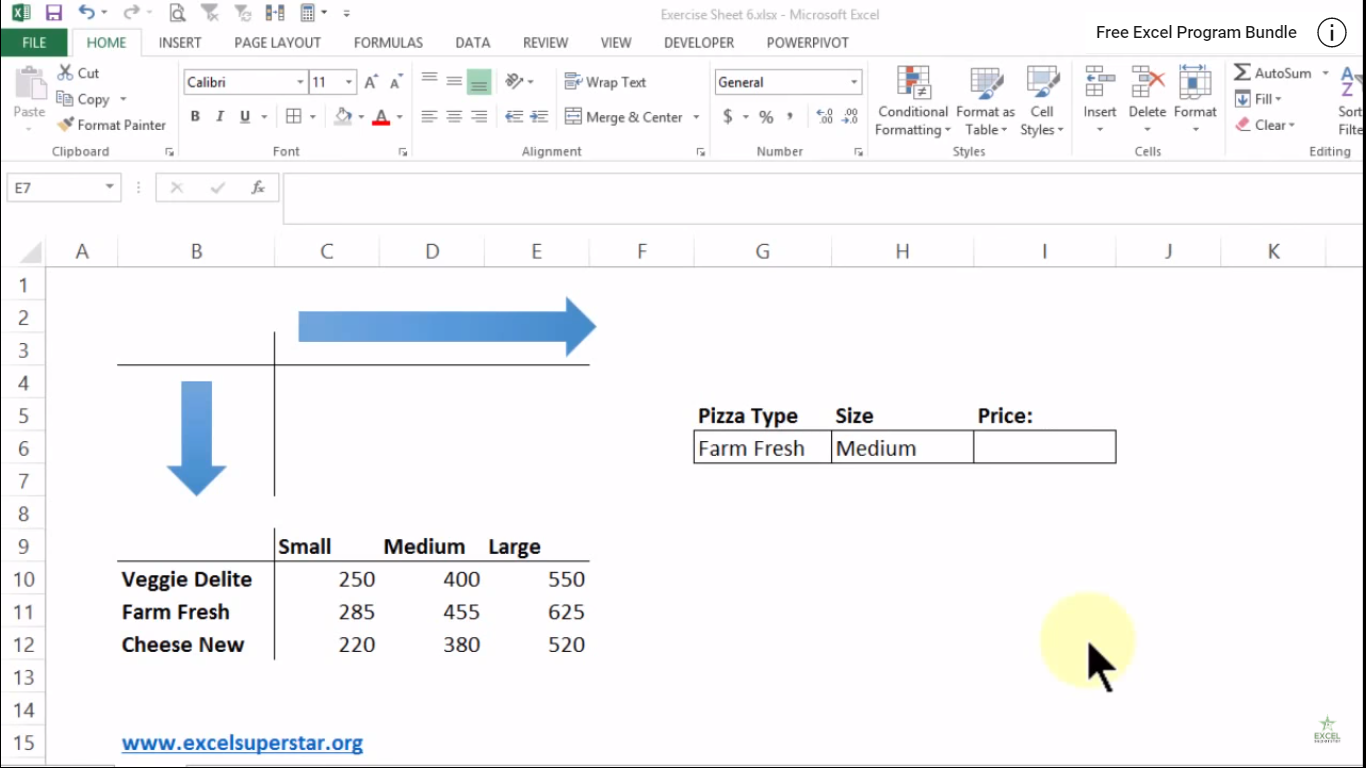I have a table containing a series of Pizza topics vertically from top to bottom along with the prices of different sizes of Pizza like Small, Medium, Large.

Now the user wants to find out the price of Medium Farm House Pizza. So how to find it?

So in order to find out the price, we will be applying VLOOKUP Formula along with the MATCH Formula by following the steps given below:

Note – We will apply this formula type only when we have two variable and want to find out the answer from the Middle

1. Write =VLOOKUP and press the Tab key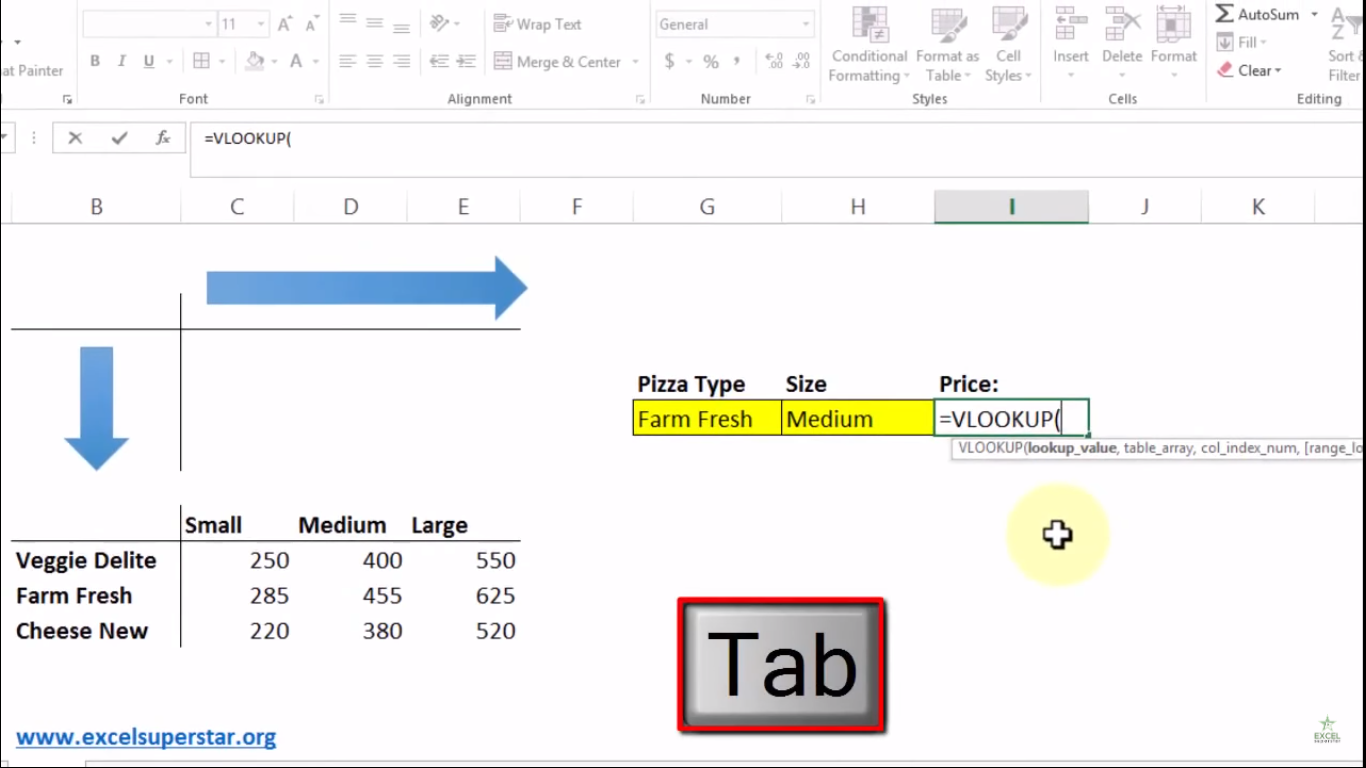Now there are 4 parameters (lookup_value, table_array, col_index_num, [range_lookup])

The first parameter lookup_value means the value to look for in the first column of a table.

2. Select Farm Fresh Pizza type as lookup_value =VLOOKUP(G6,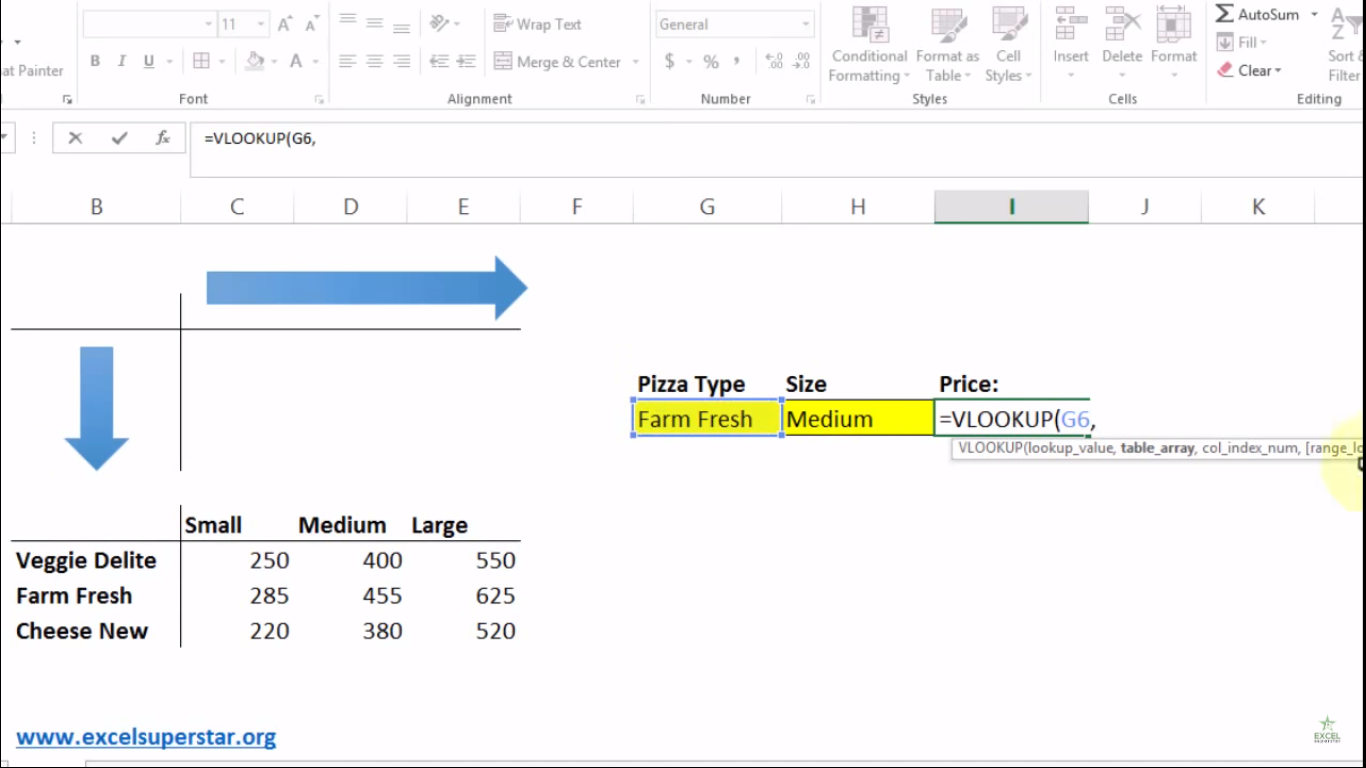The second parameter table_array means the table from which to retrieve a value.

3. Select the entire table and press F4 =VLOOKUP(G6,\$B\$9:\$E\$12,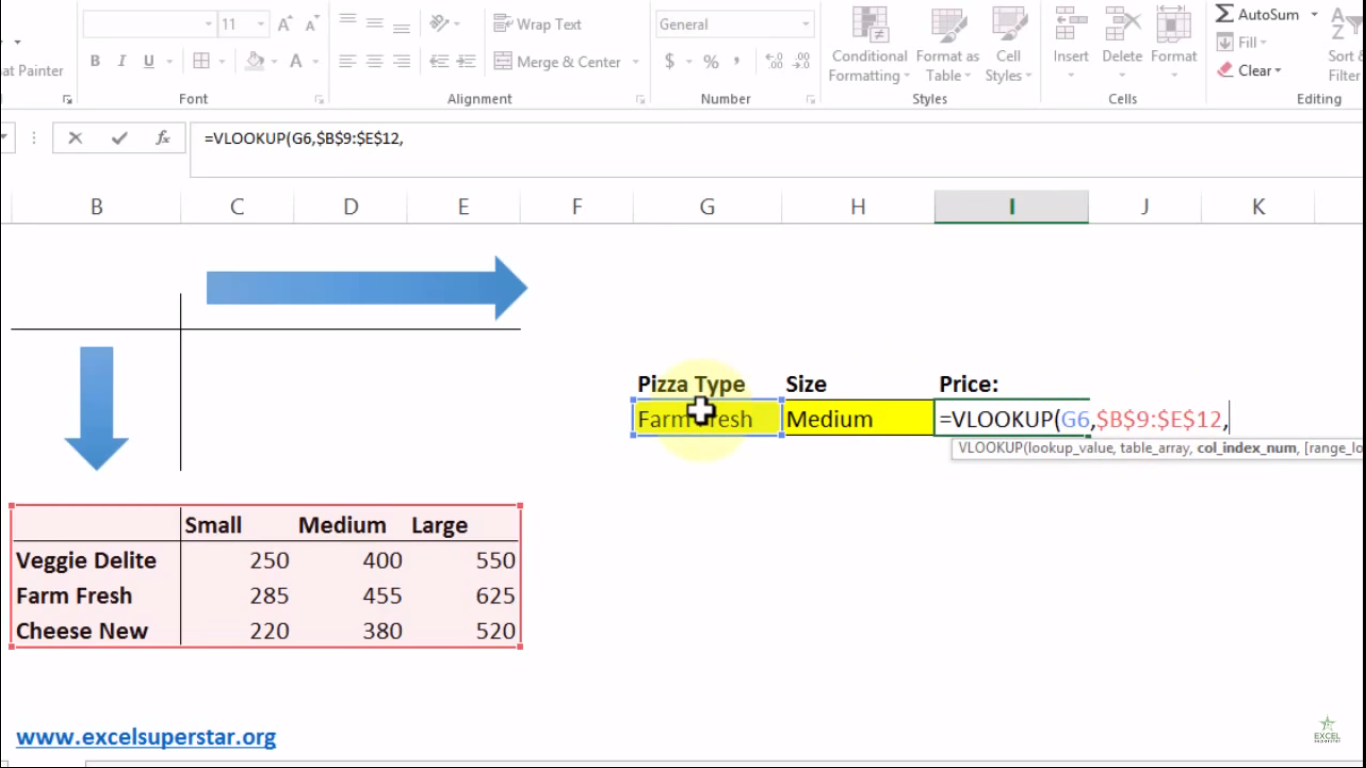The third parameter col_index_num means the column in the table from which to retrieve a value.

4. Write 3 =VLOOKUP(G6,\$B\$9:\$E\$12,3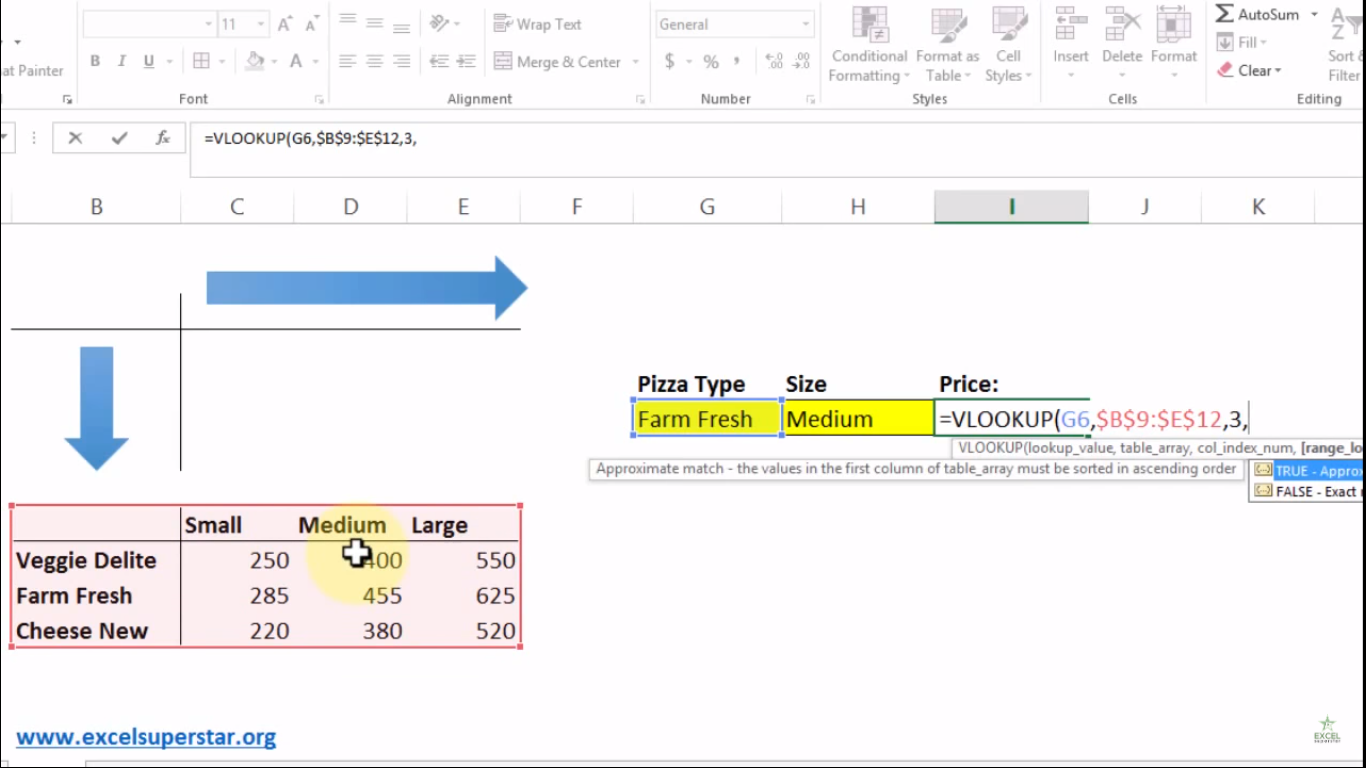Note – Here we have written the value 3 because the price of Medium Pizza lies in 3rd column

The fourth parameter range_lookup means TRUE = approximate match and FALSE = exact match.

5. Write FALSE or 0 to get the exact match of an answer and close the bracket
=VLOOKUP(G6,\$B\$9:\$E\$12,3,0)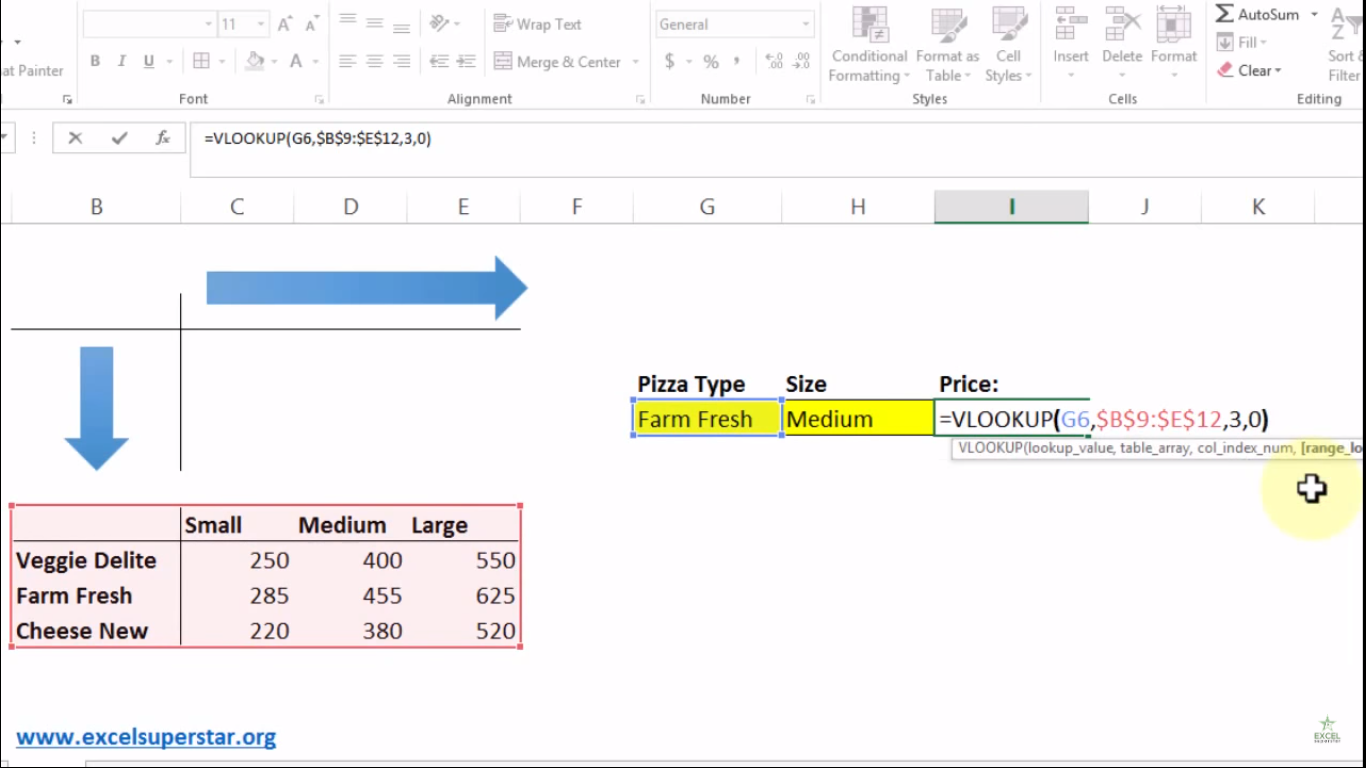6. Press Enter and you will get 455 which is the price of Medium Size Farm Fresh Pizza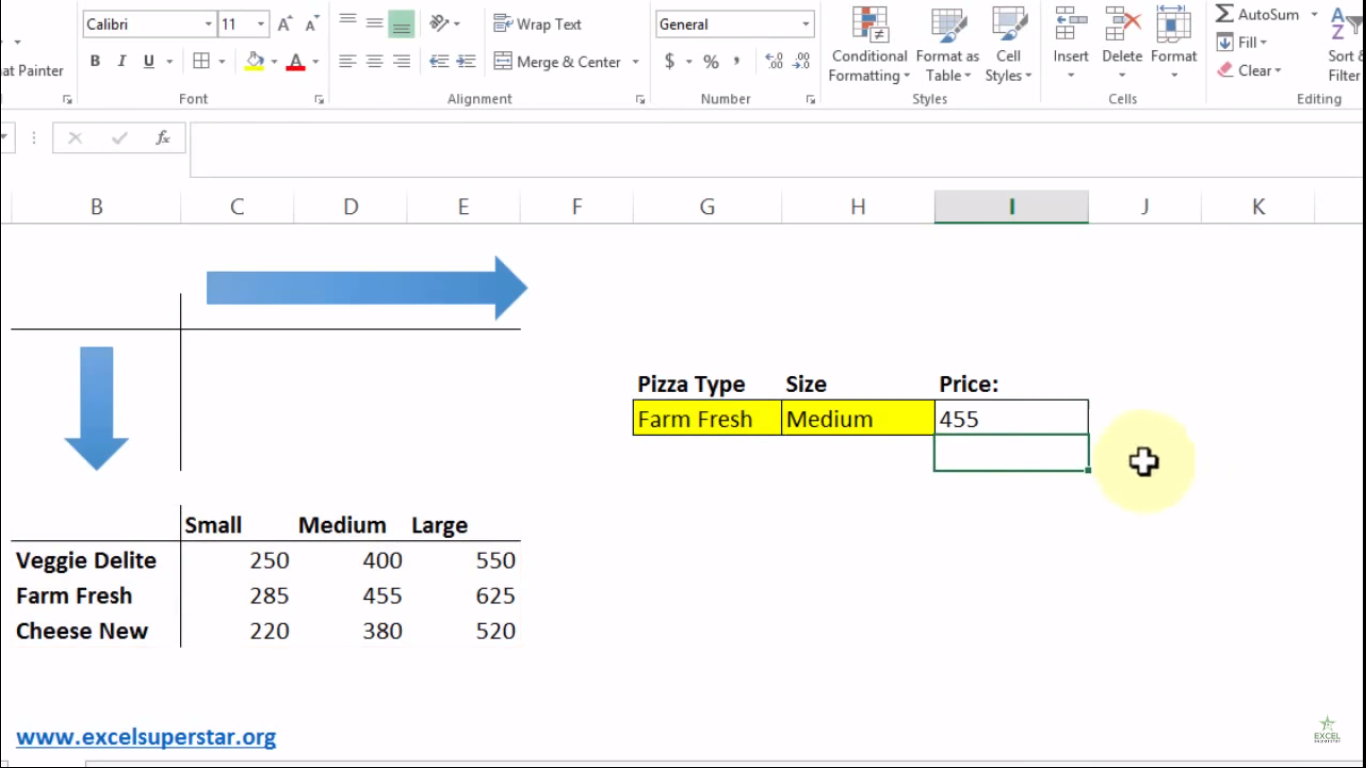Now, if I change the size and type of Pizza from Medium to Small and Farm Fresh to Veggie Delight, then the size will not change. So inside the VLOOKUP Formula, we will replace the col_index_num parameter and apply Match Formula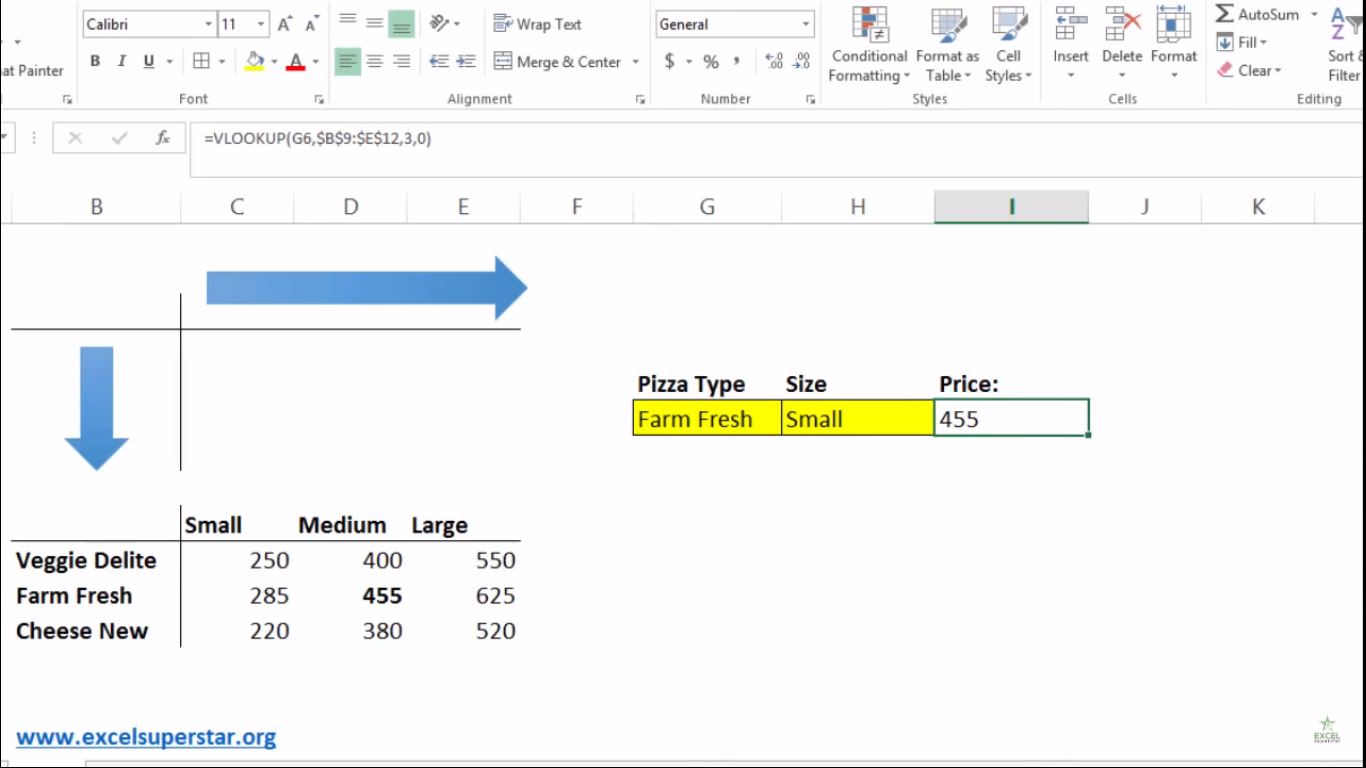Note – By applying the MATCH formula, whenever we will change the size and type of pizza, it will indicate to us in which column does the size lies and what is the price of Pizza type in the table

For The Size of the Pizza

1. Write =MATCH and press Tab Key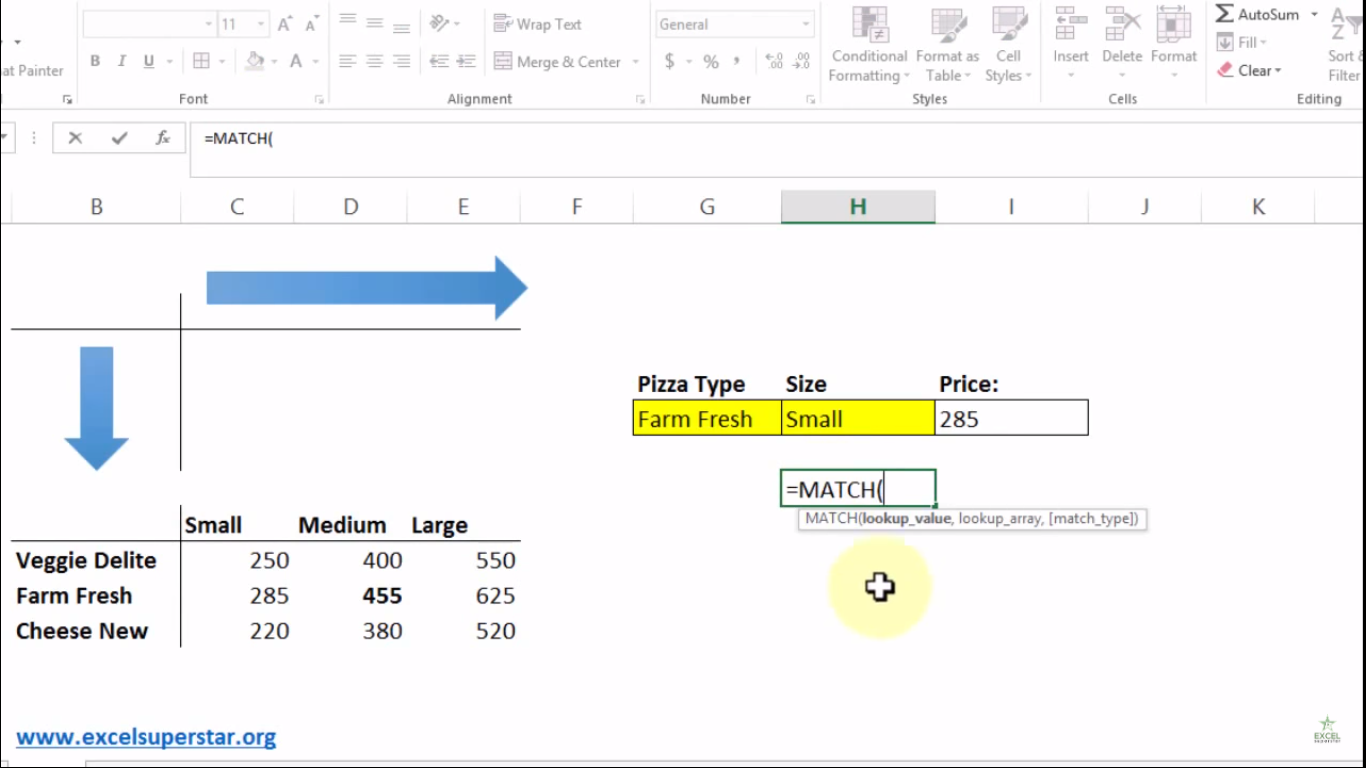Now there are 3 parameters (lookup_value, lookup_array, [match_type])

The first parameter lookup_value means the value to match in lookup_array.

2. Choose Small Size as lookup_value =MATCH(H6,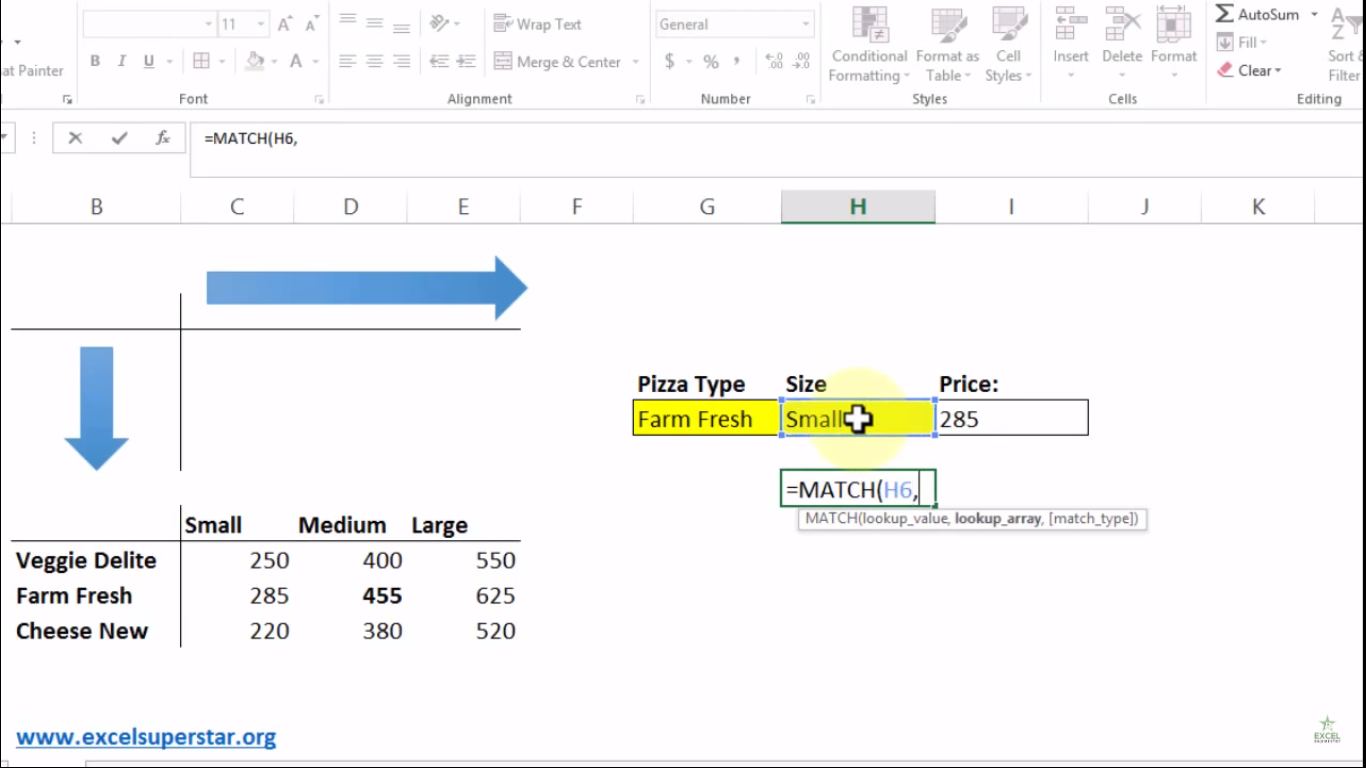The second parameter lookup_array means range of cells

3. Select the headers from the table and press F4 =MATCH(H6,\$B\$9\$E\$9,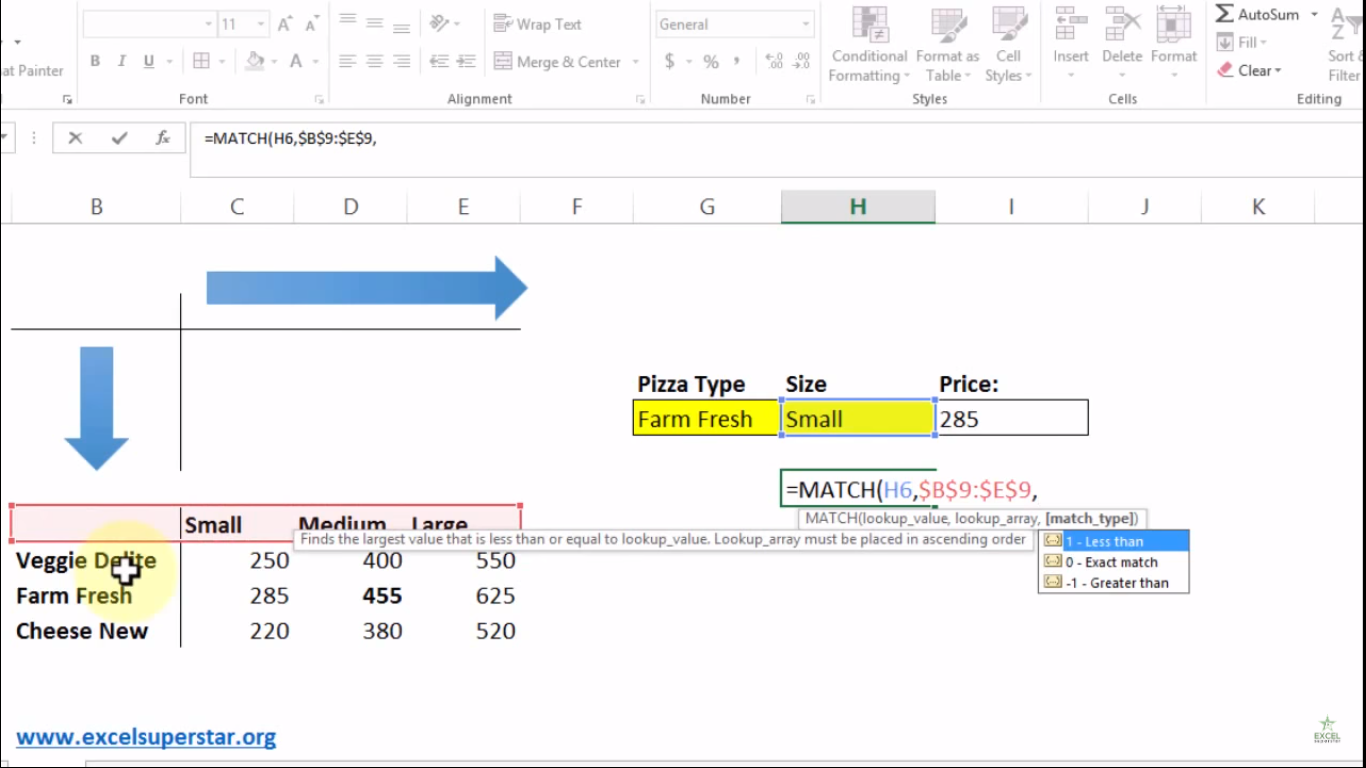The third parameter match_type means 1 =less than 0 = exact match, -1 = greater than

4. Write 0 as exact match and close the bracket =MATCH(H6,\$B\$9\$E\$9,0)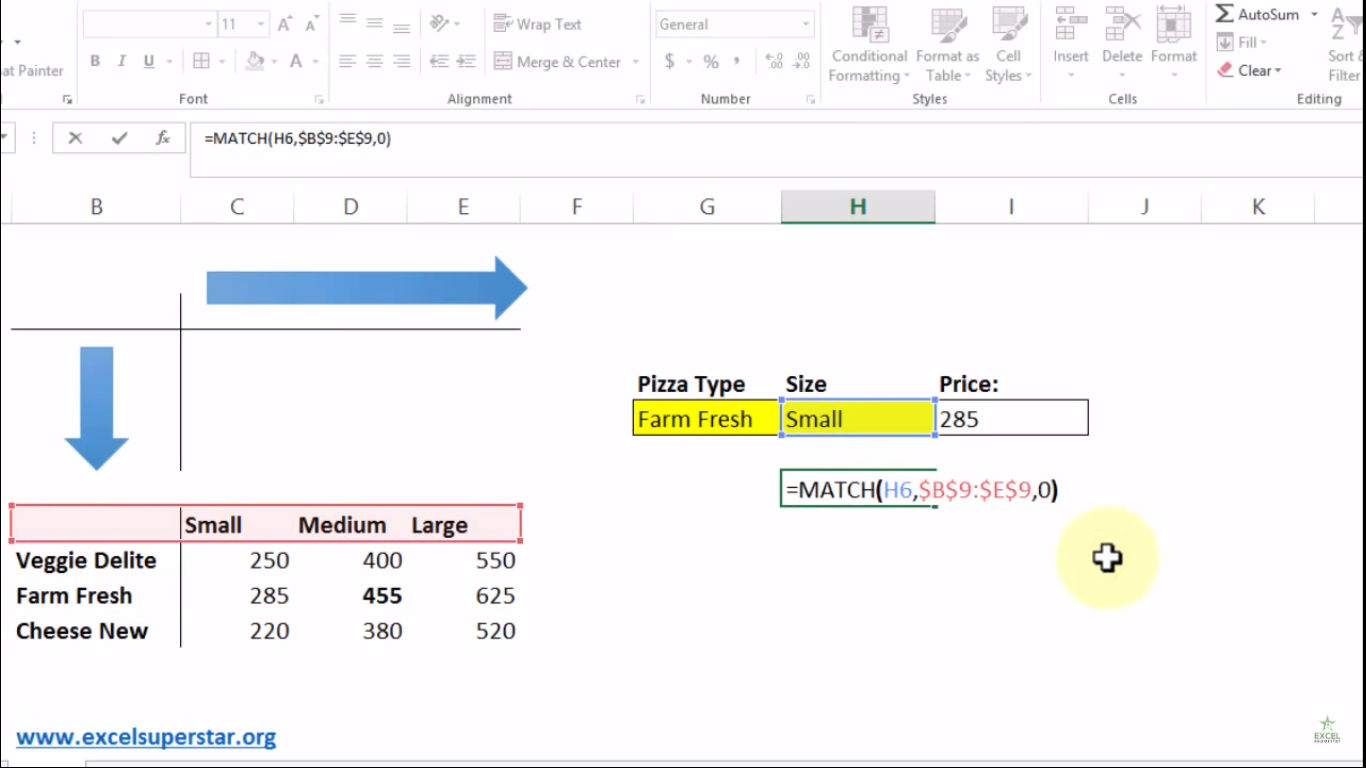5. Lastly press Enter and the answer is 2. So whenever you will change the size of the pizza you will get the answer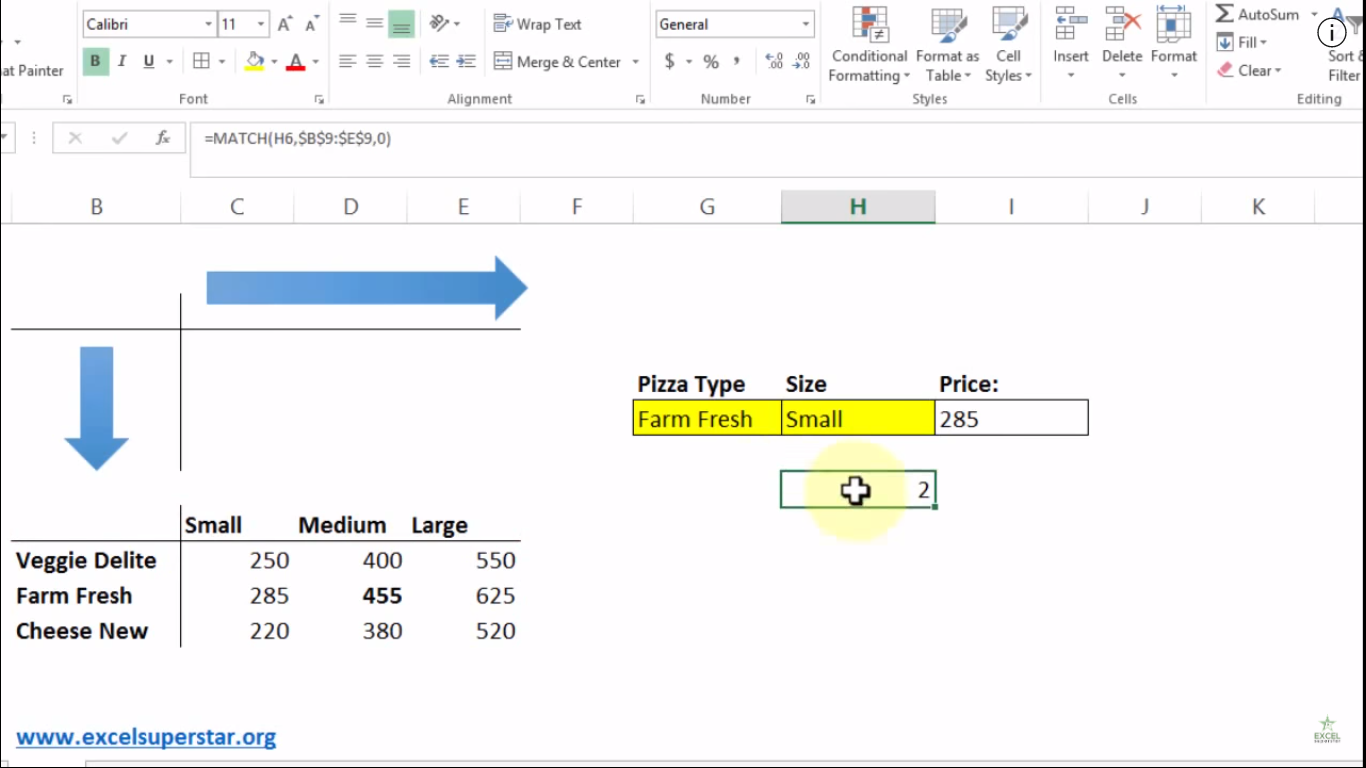For Pizza Type

Now inside the VLOOKUP formula we will replace the col_index_num and apply the MATCH Formula

1. Write MATCH and reserve the seat by opening and closing the bracket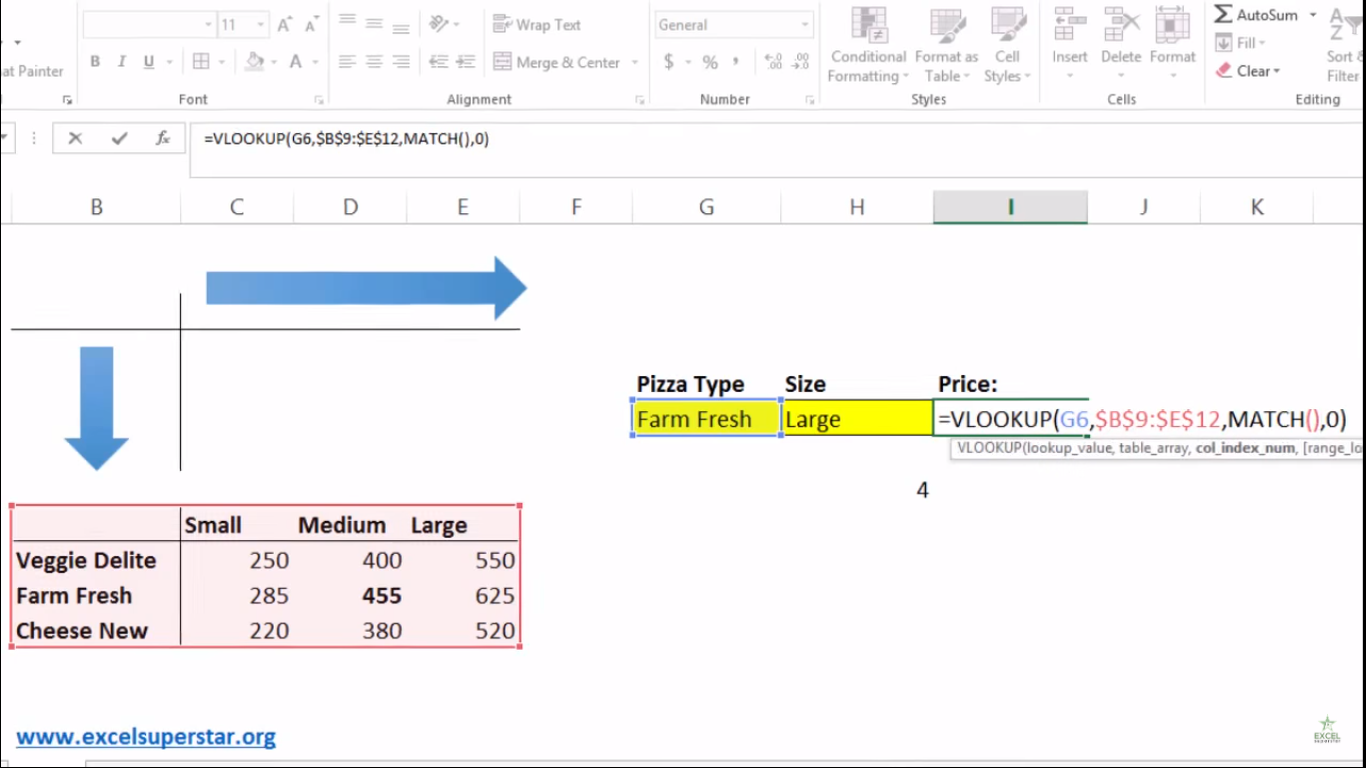2. Choose Small Size as lookup_value MATCH(H6,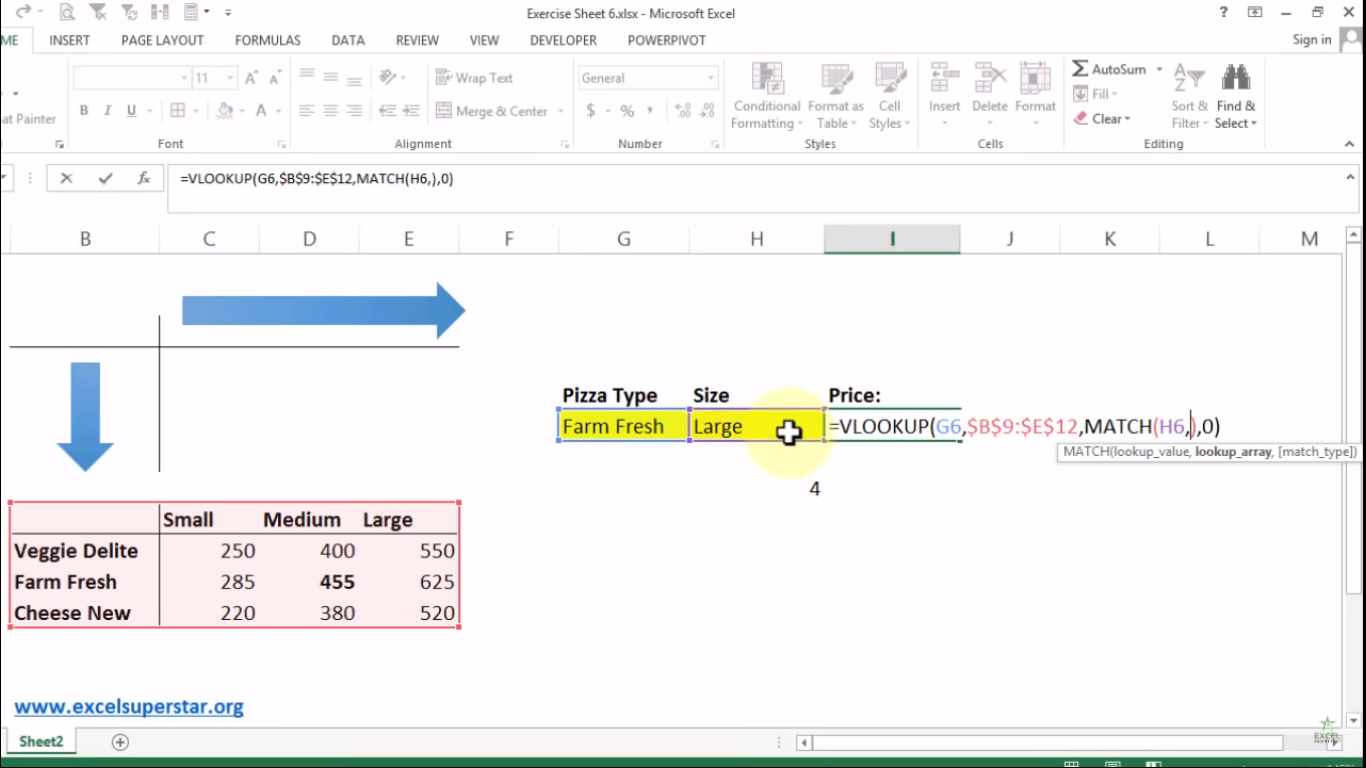3. Select the headers from the table and press F4 MATCH(H6,\$B\$9\$E\$9,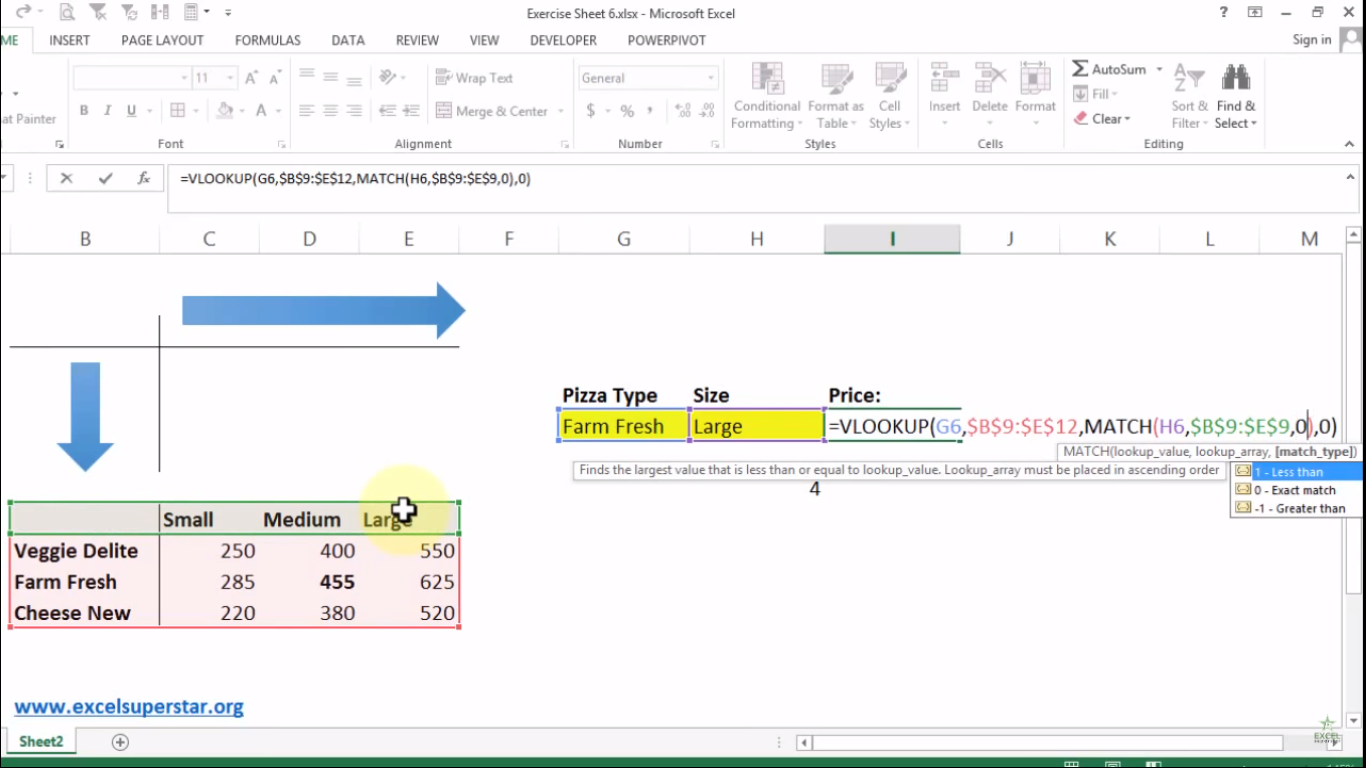4. Write 0 as exact match and close the bracket MATCH(H6,\$B\$9\$E\$9,0)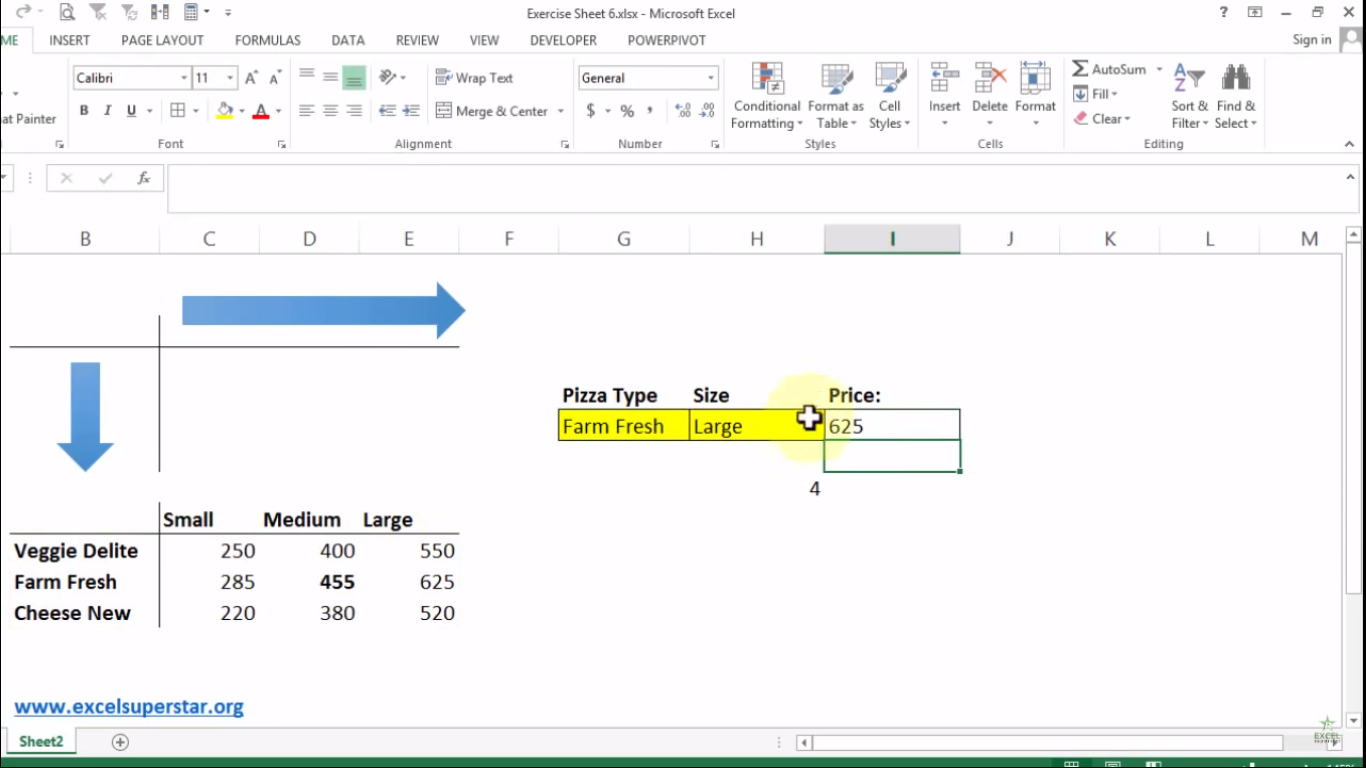5. Lastly press Enter and the answer is 2.